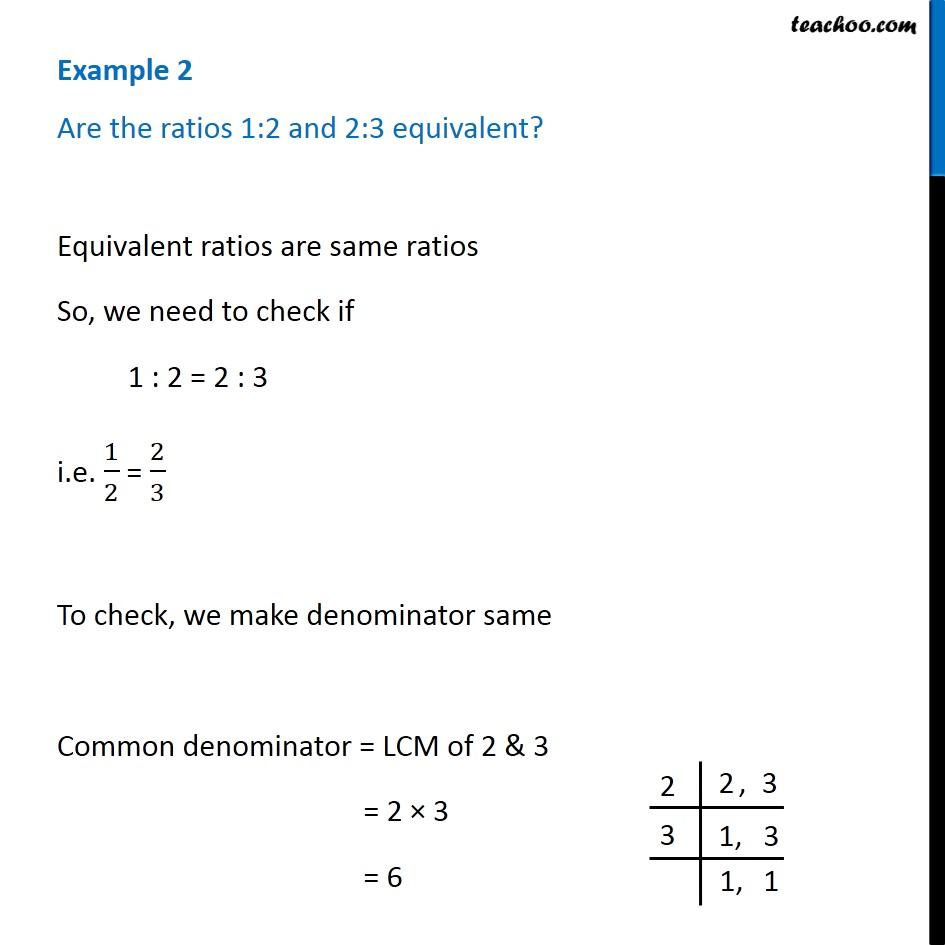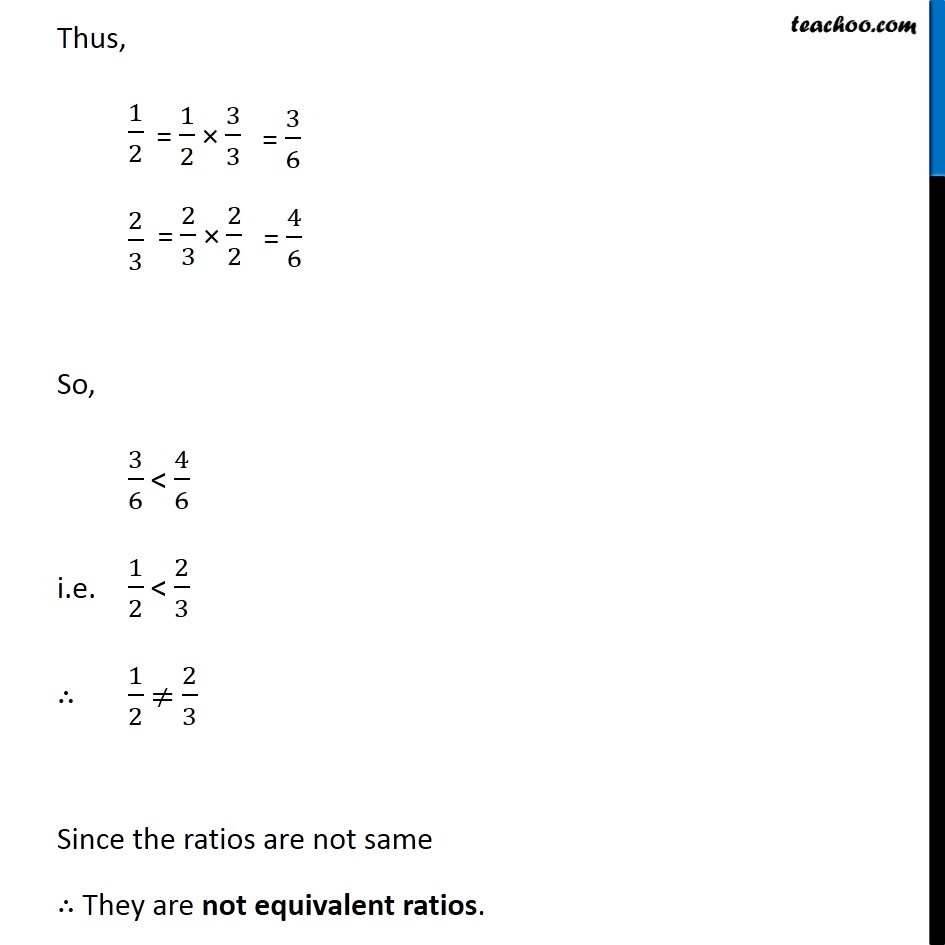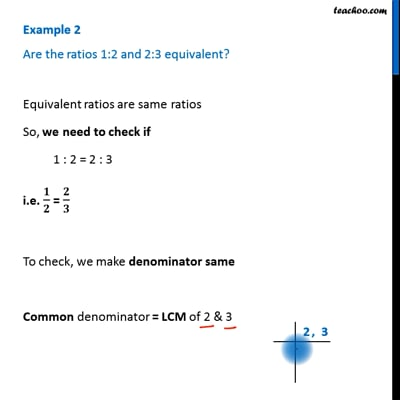Examples

Chapter 7 Class 7 Comparing Quantities
Serial order wiseThis video is only available for Teachoo black users

Learn in your speed, with individual attention - Teachoo Maths 1-on-1 Class

### Transcript

Question 2 Are the ratios 1:2 and 2:3 equivalent? Equivalent ratios are same ratios So, we need to check if 1 : 2 = 2 : 3 i.e. 1/2 = 2/3 To check, we make denominator same Common denominator = LCM of 2 & 3 = 2 × 3 = 6 Thus, 1/2 2/3= 1/2 × 3/3 = 3/6 2/3 × 2/2 = 4/6 = 4/6